Connect with us

Process Costing [An Easy Guideline]Published

on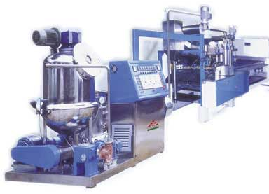Process costing is applicable to a continuous process of production of the same or similar goods, for example, oil refining and chemical production. Since the product is uniform, there is no need to determine the costs of different groups of products and each processing department becomes a cost center. This post simply demonstrates an easy way to understand concept of the process costing. Through a case example presented here, you will learn how to compute it. Enjoy!

Process costing computations can be broken down into the 5 steps listed below:

1. Visualize the physical flow of units
2. Compute the equivalent units of production
3. Determine costs to allocate
4. Compute unit costs
5. Allocate total costs to: (a) Gods Completed (b.)Ending work in process

Note that the five steps above can be memorized using the acronym: PECUA (Physical Flow, Equivalent Units of Production, Costs to Allocate, Unit Costs, Allocate Costs) which are described next.

Flow of Units

The cost flow diagram shown under Section B in this module is the same for process costing except there will typically be several WIP accounts (i.e., one for every department). When solving a process costing problem, it is helpful to visualize the physical flow of units, as illustrated in the diagram below.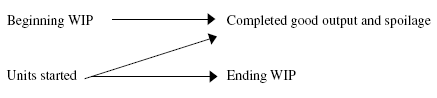The units in the beginning work-in-process [BWIP] are either completed or become spoiled. Units started during the period but not completed become the ending work-in-process [EWIP].

Equivalent Units of Production [EUP]

An EUP is the amount of work equivalent to completing one unit from start to finish. In a process costing system, products are assigned costs periodically [usually monthly]. At any one moment some units are incomplete which makes the EUP calculations necessary to allocate manufacturing costs between:

• Goods finished during the period (cost of goods manufactured)
• Ending work in process

The two primary EUP methods used for process costing are first-in, first-out [FIFO] and weighted average [WA]. Under the weighted-average approach, current costs are combined with prior period costs, and all units are carried at an average cost of production. Importantly, the method assumes that all units completed during a period are started and completed during that period. As a result, the percentage of work done last period on the beginning work in process inventory is ignored.

Process Costing Case Example

The Lie Dharma Putra Company uses a weighted-average process cost system to collect costs. Data relevant to 2009 production is given below. Assume we begin with 800 units 25% complete for labor and overhead (conversion costs), and 100% complete for materials because they are introduced at the start of the process. We start 4,200 units. 4,000 units are completed, while 1,000 remain in Ending WIP (20% complete for labor and overhead and 100% complete for materials). No spoilage exists.

The costs are summarized in the following T-account: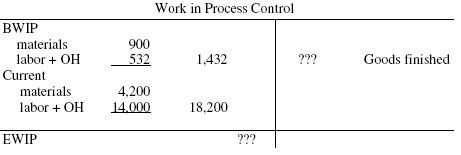Step 1: The physical flow of units is accounted for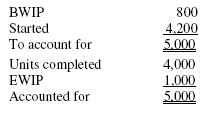Step 2: The units completed and ending work in process are converted to equivalent units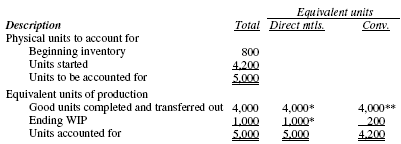Notes:

* These units are 100% complete with respect to materials because materials are introduced at the start of the process.
** These units are 100% complete with respect to conversion because all units completed are assumed to be started and completed during the period.

Step 3 and 4: Determine costs to allocate and equivalent unit costs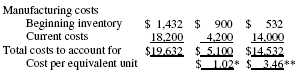Notes:

* Notice the resulting costs are averages: \$5,100 / 5,000 equivalent units = \$1.02.
** \$14,532 / 4,200 equivalent units = \$3.46.

Step 5: Allocate total costs to goods completed and ending work in process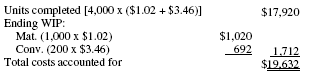The allocation is accomplished by multiplying the individual equivalent unit figures by the unit costs.

Equivalent Unit production for Material

In the above example, material was assumed to be added at the beginning of the production process. Material can also be added at different points in the process (e.g., 10%, 70%) or gradually during the process.

FIFO Work in Process Assumption

With FIFO, the first batch into production (i.e., the beginning work in process inventory) is assumed to be the first batch completed. This batch is treated as a separate, distinct layer—separate from goods that are started and completed during the period.

The weighted-average assumption (all goods are assumed to be started and completed during the period) no longer holds for FIFO. Thus, any work done last period on the beginning work in process inventory must be taken into consideration. After all is said and done, the equivalent unit figures reflect the work done during the current accounting period. Also, the only difference between the two methods is the treatment of the beginning work in process inventory.
The equivalent-unit calculations for Lie Dharma Putra Company follow: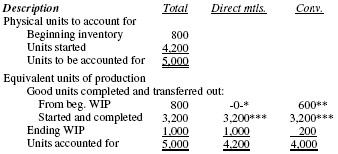Notes:
* All material was introduced last period.
** 75% of the work was necessary this period to complete the units.
*** 100% of the materials and conversions were introduced this period.

Spoilage (Scrap) in Process Costing

The following terms are commonly used:

• Spoilage (scrap)—Inferior goods either discarded or sold for disposal value
• Defective units—Inferior goods reworked and sold as normal product

A major distinction is made between normal and abnormal spoilage:

• Normal spoilage is the cost of spoiled units caused by the nature of the manufacturing process (i.e., which occur under efficient operating conditions). Normal spoilage is a necessary cost in the production process and is, therefore, a product cost. It is a period cost (e.g., “loss on abnormal spoilage”).
• Abnormal spoilage is the cost of spoiled units which were spoiled through some unnecessary act, event, or condition. It is costs should not be included in cost of goods sold.

Spoilage must be considered in EUP calculations. For example: if spoilage is discovered at the 60% point in processing and 100 units of abnormal spoilage are discovered, 60 EUP have occurred. The amount of abnormal loss would be the cost of 60 EUP (processing) plus the materials added to 100 units of production up to the 60% point. In contrast, if the spoilage was considered normal in nature, the spoilage cost would be treated as a product cost and simply added to the cost of the good units completed.

Are you looking for easy accounting tutorial? Established since 2007, Accounting-Financial-Tax.com hosts more than 1300 articles (still growing), and has helped millions accounting student, teacher, junior accountants and small business owners, worldwide.